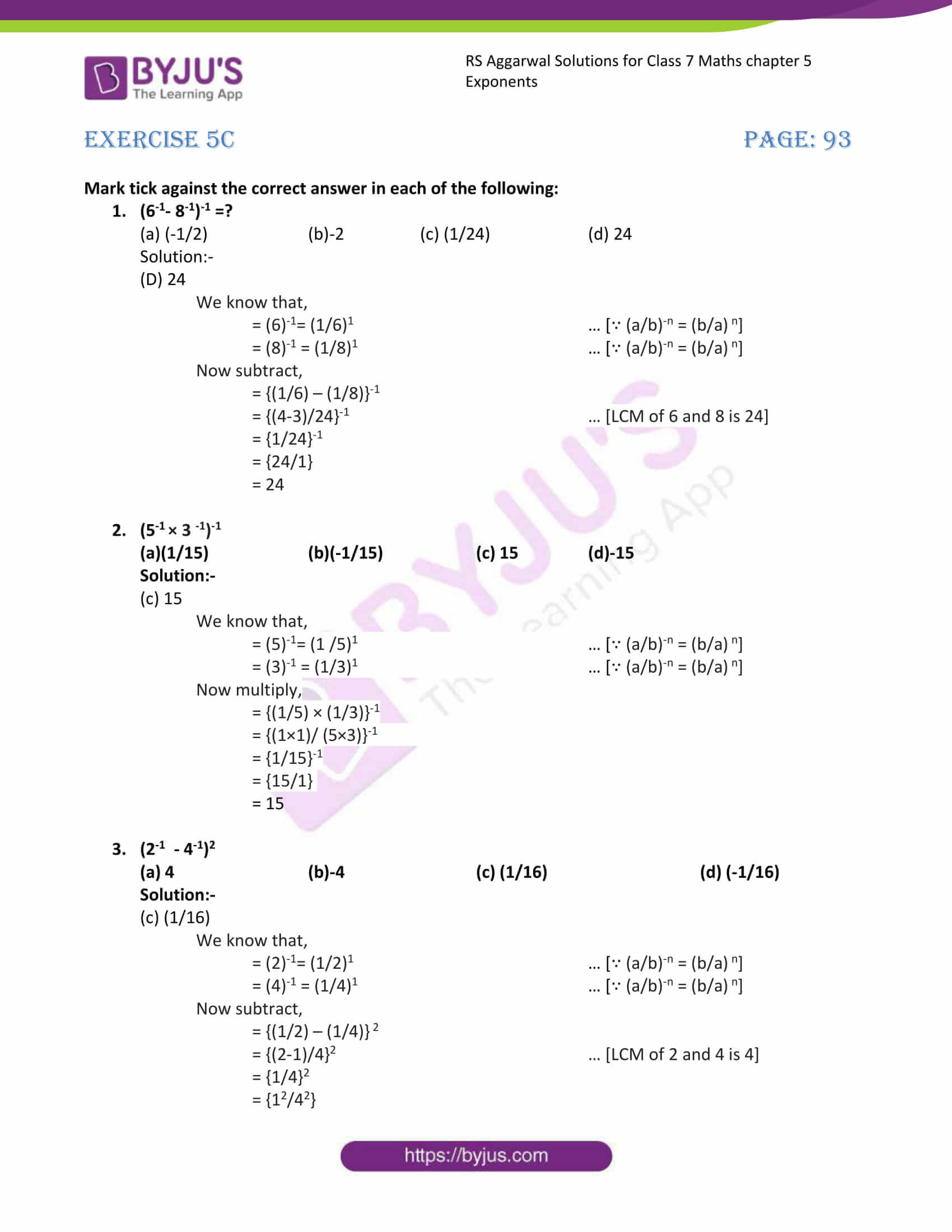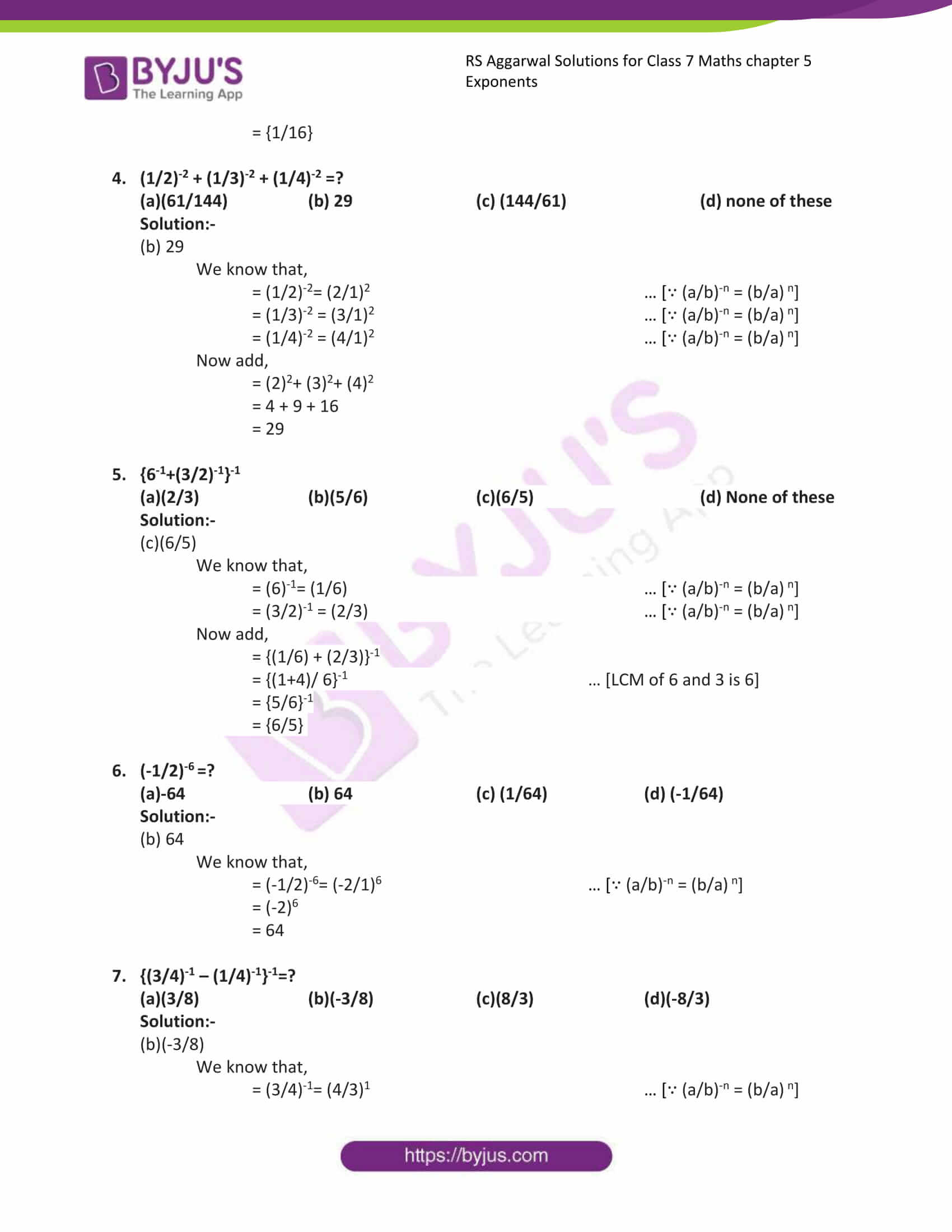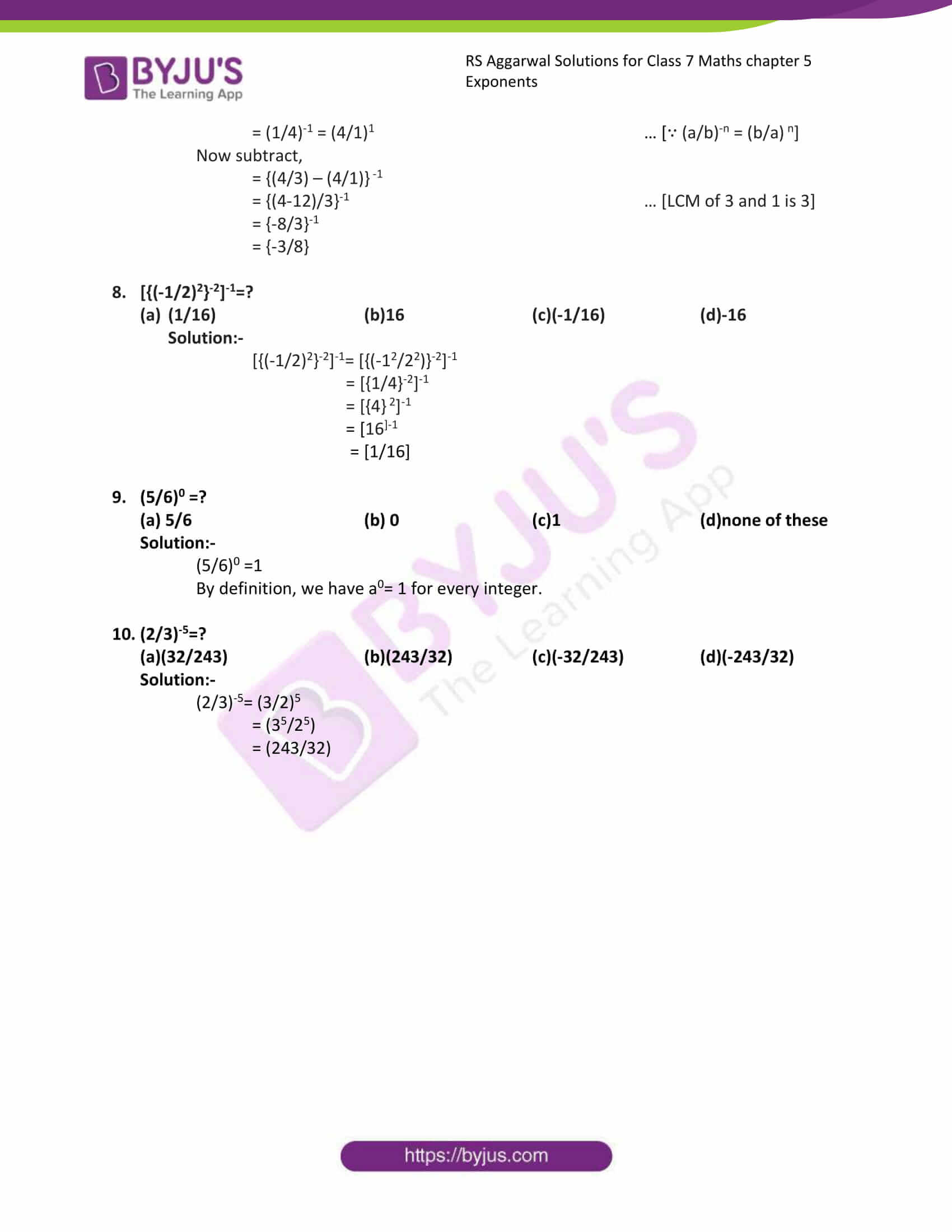# RS Aggarwal Solutions for Class 7 Maths Exercise 5C Chapter 5 Exponents

RS Aggarwal Solutions For Class 7 Maths Chapter Exercise 5C Chapter 5 Exponents has objective type questions. In this exercise, application-level questions are covered to make the students think in various ways to get the answer. The objective type questions in this exercise which are based on all the topics covered in this chapter. Our expert teachers formulate these RS Aggarwal Solutions For Class 7 Maths Chapter 5 Exponents. To help you with your exam preparation to achieve good marks in Maths Students are advised to try solving the problems from RS Aggarwal Solutions book of Class 7. Then refer to these solutions to know the best way of approaching the different questions.

## Download the PDF of RS Aggarwal Solutions For Class 7 Maths Chapter 5 Exponents – Exercise 5C### Access answers to Maths RS Aggarwal Solutions for Class 7 Chapter 5 – Exponents Exercise 5C

Mark tick against the correct answer in each of the following:

1. (6-1– 8-1)-1 =?

(a) (-1/2) (b)-2 (c) (1/24) (d) 24

Solution:-

(D) 24

We know that,

= (6)-1= (1/6)1 … [∵ (a/b)-n = (b/a) n]

= (8)-1 = (1/8)1 … [∵ (a/b)-n = (b/a) n]

• Now subtract,
• = {(1/6) – (1/8)}-1
• = {(4-3)/24}-1 … [LCM of 6 and 8 is 24]

= {1/24}-1

= {24/1}

= 24

2. (5-1 × 3 -1)-1

(a)(1/15) (b)(-1/15) (c) 15 (d)-15

Solution:-

(c) 15

We know that,

= (5)-1= (1 /5)1 … [∵ (a/b)-n = (b/a) n]

= (3)-1 = (1/3)1 … [∵ (a/b)-n = (b/a) n]

Now multiply,

= {(1/5) × (1/3)}-1

= {(1×1)/ (5×3)}-1

= {1/15}-1

= {15/1}

= 15

3. (2-1 – 4-1)2

(a) 4 (b)-4 (c) (1/16) (d) (-1/16)

Solution:-

(c) (1/16)

We know that,

= (2)-1= (1/2)1 … [∵ (a/b)-n = (b/a) n]

= (4)-1 = (1/4)1 … [∵ (a/b)-n = (b/a) n]

Now subtract,

= {(1/2) – (1/4)} 2

= {(2-1)/4}2 … [LCM of 2 and 4 is 4]

= {1/4}2

= {12/42}

= {1/16}

4. (1/2)-2 + (1/3)-2 + (1/4)-2 =?

(a)(61/144) (b) 29 (c) (144/61) (d) none of these

Solution:-

(b) 29

We know that,

= (1/2)-2= (2/1)2 … [∵ (a/b)-n = (b/a) n]

= (1/3)-2 = (3/1)2 … [∵ (a/b)-n = (b/a) n]

= (1/4)-2 = (4/1)2 … [∵ (a/b)-n = (b/a) n]

= (2)2+ (3)2+ (4)2

= 4 + 9 + 16

= 29

5. {6-1+(3/2)-1}-1

(a)(2/3) (b)(5/6) (c)(6/5) (d) None of these

Solution:-

(c)(6/5)

We know that,

= (6)-1= (1/6) … [∵ (a/b)-n = (b/a) n]

= (3/2)-1 = (2/3) … [∵ (a/b)-n = (b/a) n]

= {(1/6) + (2/3)}-1

= {(1+4)/ 6}-1 … [LCM of 6 and 3 is 6]

= {5/6}-1

= {6/5}

6. (-1/2)-6 =?

(a)-64 (b) 64 (c) (1/64) (d) (-1/64)

Solution:-

(b) 64

We know that,

= (-1/2)-6= (-2/1)6 … [∵ (a/b)-n = (b/a) n]

= (-2)6

= 64

7. {(3/4)-1 – (1/4)-1}-1=?

(a)(3/8) (b)(-3/8) (c)(8/3) (d)(-8/3)

Solution:-

(b)(-3/8)

We know that,

= (3/4)-1= (4/3)1 … [∵ (a/b)-n = (b/a) n]

= (1/4)-1 = (4/1)1 … [∵ (a/b)-n = (b/a) n]

Now subtract,

= {(4/3) – (4/1)} -1

= {(4-12)/3}-1 … [LCM of 3 and 1 is 3]

= {-8/3}-1

= {-3/8}

8. [{(-1/2)2}-2]-1=?

(1/16) (b)16 (c)(-1/16) (d)-16

Solution:-

[{(-1/2)2}-2]-1= [{(-12/22)}-2]-1

= [{1/4}-2]-1

= [{4} 2]-1

= -1

= [1/16]

9. (5/6)0 =?

(a) 5/6 (b) 0 (c)1 (d)none of these

Solution:-

(5/6)0 =1

By definition, we have a0= 1 for every integer.

10. (2/3)-5=?

(a)(32/243) (b)(243/32) (c)(-32/243) (d)(-243/32)

Solution:-

(2/3)-5= (3/2)5

= (35/25)

= (243/32)

### Access other exercises of RS Aggarwal Solutions For Class 7 Chapter 5 – Exponents

Exercise 5B Solutions